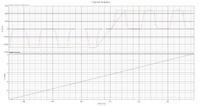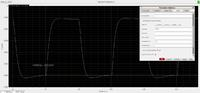# Monte Carlo anlaysis to find Vth mismatch

Status
Not open for further replies.
When I plotted histogram of Vth of one of the transistors,It is showing a standard deviation of 6.5m and mean of 283m.Does this mean Vth mismatch(and hence the offset of comparator) will be around 19.5mV(3*6.5)??

When I am calculating the offset of a circuit I use a slow ramp input voltage close to 0 (differential)
I run a transient analysis via monte carlo and I calculate the input voltage when the output crosses zero.

"Value" of "input voltage" when "cross" of "output" "0" (value and cross are the calculator functions that I use)

Keep in mind that the mean value of the offset will not be right unless you use a slow ramp (until the input is processed by the comparator the input voltage changes a bit)

•Ravinder487

### Ravinder487

Points: 2
Before comparison output of comparator will be at Vdd.
Sorry I don't understand this
I calculate the input voltage when the output crosses zero.

Before comparison output of comparator will be at Vdd.

Could you post the output and input of the comparator?

Sorry I don't understand this
I mean that in order to calculate the input referred offset you need (by definition) to see what input voltage causes the output to be zero.

•Ravinder487

### Ravinder487

Points: 2

To calculate the offset of a comparator with a reset (precharge - track, what ever) I strobe the output to get only the evaluated data.

For example, this is the output/input of track-and-latch comparator. If I were to check when the output crosses zero, I would get wrong results. So I strobe the output to get only the data I want. (the gray output, superimposed on the real one, is strobed)Here is the strobing parameters I used above.
I have set the clock period at 1ns -> strobing period 1ns for this circuit.
I have also set the skipstart at .5ns in order to start strobing when the input is already evaluated.•Ravinder487

### Ravinder487

Points: 2
Hi Lamoun,
I doubt, your method gives me the resolution of comparator not the offset of comparator,correct me if I'm wrong

With a really slow ramp you could estimate the resolution of the comparator.

But running monte carlo analysis with the equation I mention above gives you the offset of the comparator.

•Ravinder487

### Ravinder487

Points: 2
That sounds great,I will do the method you suggested and will let you if there are any discrepancies!!

Plotting Vth does not make sense anyway - Vth i susually the parameter to be spread by monte carlo.

My idea is to cancel offset of comparator using body biasing of driving transistors in sense amplifier.The method is explained in Pg.No.490 of following attachment

#### Attachments

• comparator-sensor.pdf
861 KB · Views: 129

Status
Not open for further replies.